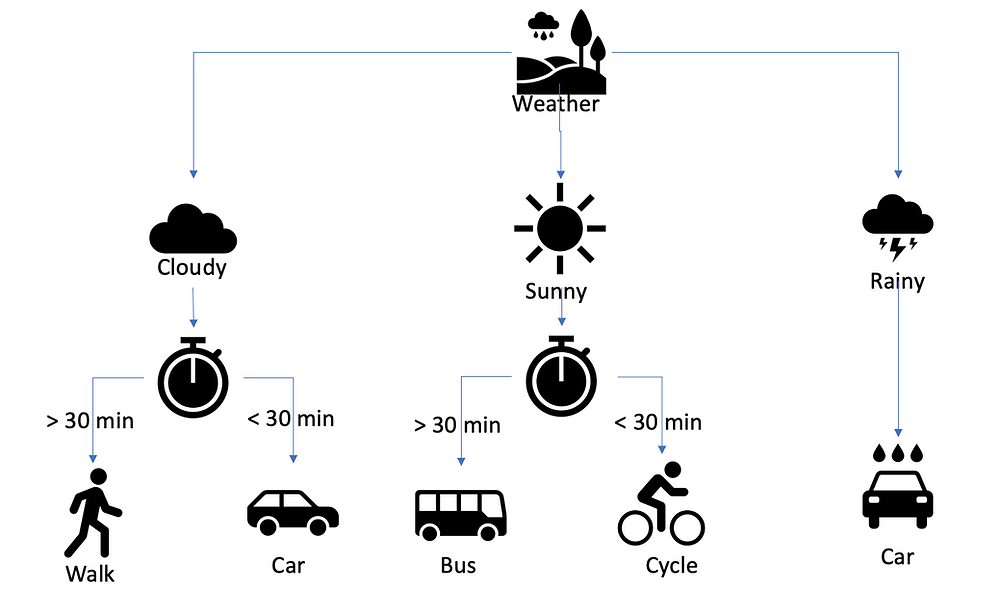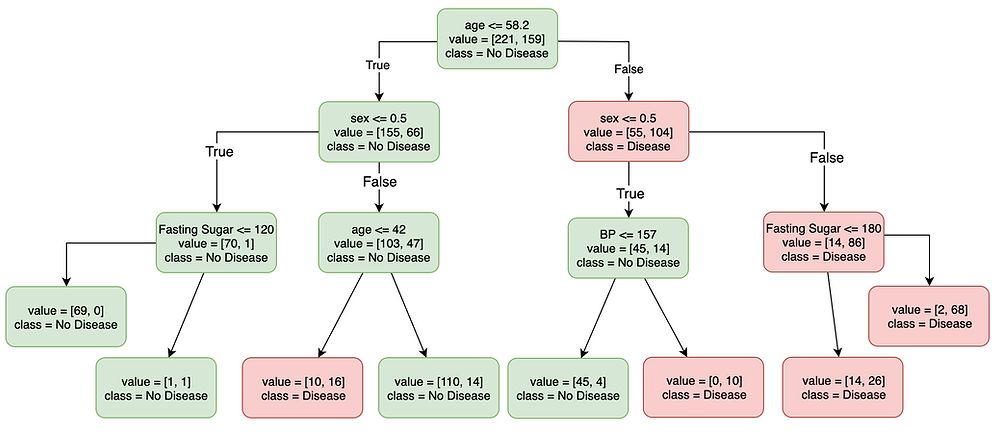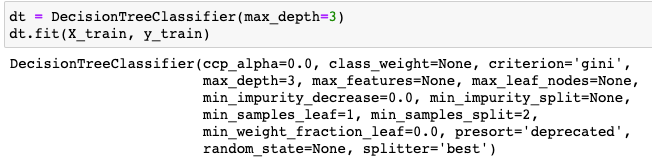Search
•Sai Geetha M N

# Decision Trees - An Introduction

Decision trees are an algorithm class that form the foundation for Random Forests, a class of algorithms that is extensively used in classification but can be used for regression too. This is a non-linear model, unlike linear or logistic regression models that we have seen so far.

## What is a Decision Tree?

A decision tree algorithm is one that mimics the human decision-making process. It checks an answer to a question which can have more than one answer, branches off based on an answer and then the next question is asked. This continues till all questions are answered.

For example, taking a very simplistic situation, which we mentally sort out very easily on a daily basis – taking a decision based on weather and time available as to what transport I should choose to reach a venue, will look like the below decision tree:Is the Weather cloudy, sunny or rainy? And then the next question answered is the amount of time available to reach the destination. Based on these two questions, a decision is made on the transportation to take.

This is clearly an oversimplification of how the decision tree works. But this is just to explain what decision trees look like.

They have root nodes – the weather node here. Then, they have internal nodes like the cloudy and sunny nodes. And then you have the leaf nodes that give the final decision. There is a decision made at every node that decides the direction of the final decision.

Decision trees are clearly supervised models built based on already existing data that help create the internal and final leaf nodes based on various criteria.

As you can see it is a highly interpretable model. If you reach the decision to walk, you know it is because it is cloudy and you have more than 30 minutes to reach the venue.

Let us see a practical example, of a model that helps in predicting whether one is diabetic or not:Here you can see that people aged less than 58 and are females with fasting sugar less than 120 – mostly do not have diabetes. And people above 58 and males with fasting sugar <= 180 mostly have diabetes. There are many intermediate stages where the decision might change to having or not having diabetes. Also, a majority class at that node decides the class of that node bringing in only a particular level of accuracy in the prediction.

To get more and more accurate, maybe you go on till every node has only one data point and that is accurately predicted. This would then be a complete case of overfitting with 100% accuracy on the train data and may perform very poorly on any test data. This has to be avoided by finetuning what are called hyperparameters that are discussed later.

#### Points to note about the algorithm:

1. It is a supervised algorithm – meaning that it learns from already classified data or data which already has the variable that needs to be predicted

2. It is also called a greedy algorithm. It maximises the immediate benefit rather than taking a holistic approach. The greedy approach makes it vary drastically with even small variations in the data set. Hence, it is called a high variance model.

3. This happens in a top-down approach as well.

## How does it work?

As you can see, we recursively split the data into smaller data sets. Based on what? Based on some features and the values of those features.

The data has to be split in such a way that the homogeneity or the purity of the subset created should be maximised. However, how do we decide which feature to split by, first and which feature goes next? How do we decide what is the value based on which the split threshold is decided? How long do we go on splitting i.e. what is the stopping criterion?

There are what are called Hyperparameters that help in making most of the decisions that need to be made.

## Hyperparameters

Hyperparameters are simple parameters that are passed to a learning algorithm to control the training of the model. This is what helps in tuning the behaviour of the algorithm.

For example, in the Decision tree model, when the model is instantiated, a hyperparameter that can be passed is the max_depth – the number of levels that you want to split and train up to.

So, if you give max_dept as 5, the splits will happen only up to 5 levels even though the accuracy may be questionable. Hence there is a lot of power in the hands of the model designer to tune the learning to get a better result.

Similarly, there are many more hyperparameters that we will see with more examples in later posts which will make this concept clearer.

Just to get a peek into the possible hyperparameters, look at this piece of code:Here the Decision tree classifier has been given one hyperparameter i.e. max_depth. the others are left as default. But the others that can be given are max_features, max_leaf_nodes, min_impurity_decrease, min_impurity_split etc.

Tuning the values of each of these can control overfitting and yet improve the accuracy of predictions on new data.

Hope this gave you a high-level introduction to decision trees.